Copyright © Michael Richmond. This work is licensed under a Creative Commons License.

# The magnitude scale

#### Why use magnitudes?

One of the most fundamental properties of a star is its brightness. Astronomers measure stellar brightness in units called magnitudes, which seem at first counterintuitive and confusing. That's because they ARE counterintuitive and confusing -- they are in large part a legacy of ancient times. However, once you get used to them, they turn out to have a few redeeming qualities.

Why do we continue to use this system? There are several of reasons:

1. The objects we study cover a huge range in apparent brightness: the brightest star visible with the naked eye is over 600 times brighter than the faintest one. The Sun is over 6 TRILLION times brighter than the faintest star visible to the naked eye. It's awkward to deal with numbers this large.
2. As you will see below, the magnitude system is logarithmic, which turns the huge range in brightness ratios into a much smaller range in magnitude differences: the difference between the Sun and the faintest star visible to the naked eye is only 32 magnitudes.
3. Over the past few hundred years, astronomers have built up a vast literature of catalogs and measurements in the magnitude system.
4. Astronomers have figured out how to use magnitudes in some practical ways which turn out to be easier to compute than the corresponding brightness ratios.

Astronomers who study objects outside the optical wavelengths -- in the radio, ultraviolet, or X-ray regimes -- do not have any historical measurements to incorporate into their work: these fields are all very recent, dating to the 1930s or later. In those regimes, measurements are almost always quoted in "more rational" systems: units which are linear with intensity (rather than logarithmic) and which become larger for brighter objects. In the radio, for example, sources are typically measured in janskys, where

```     1 Jansky  =  10^(-26)  watts / square meter / Hertz
```

A source of strength 5 Janskys is 5 times brighter than a source of 1 Jansky, just as one would expect.

#### History of stellar measurements

People in all corners of the world have looked up at the stars (after all, without television, what else could they do at night?). We have detailed records from several cultures in the Middle East; in some, priests spent years studying the motions of the stars and planets, often trying to predict events in the future. Their motives may have been ill-founded, but in some cases, they did make very good measurements of what they could see.

Hipparchus of Rhodes compiled a catalog of about 850 stars. He described the brightness of each star by placing it in one of six categories, which one could call "brighest, bright, not so bright, not so faint, faint, faintest." Later scientists used the word "magnitude" to describe these categories.

```mag-ni-tude  n.

1. a.  Greatness of rank or position: "such duties as were expected
of a landowner of his magnitude" (Anthony Powell).
b.  Greatness in size or extent: The magnitude of the flood
was impossible to comprehend.
c.  Greatness in significance or influence:
was shocked by the magnitude of the crisis.
```
From the American Heritage� Dictionary of the English Language, Fourth Edition Copyright � 2000 by Houghton Mifflin Company.

The brightest stars were assigned to "the first magnitude", just as we would call the best movies or restaurants "first rate." The next-brightest stars were called "second magnitude", and so on down to the faintest stars visible to the unaided eye, which were called "sixth magnitude."

This is the origin of the peculiar convention that

• bright stars are denoted by small magnitudes
• faint stars are denoted by large magnitudes

#### The quantitative basis for magnitudes

In the nineteenth century, astronomers devised a number of tools which allowed them for the first time to make accurate, quantitative measurements of stellar brightness. They discovered two properties of the traditional magnitude classifications:

1. each step in the magnitude scale corresponded to roughly the same ratio in stellar brightness
2. the stars traditionally called "first magnitude" were roughly one hundred times brighter than those called "sixth magnitude".

An astronomer named N. R. Pogson came up with a system which would roughly preserve the ancient magnitude values, while allowing modern scientists to extend it to more precise measurements. He proposed that the magnitude system be defined as follows: given two stars with intensity of brightness I(1), I(2), define a magnitude difference which is based on their ratio of intensities: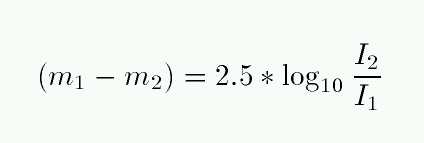So, for example,

```
intensity ratio I(1)/I(2)            magnitude difference  (m1 - m2)
----------------------------------------------------------------------
0.01                                  +5.00
0.1                                   +2.50
0.5                                   +0.75
1.0                                    0.0
2                                     -0.75
10                                     -2.50
100                                     -5.00
```

Note again the counterintuitive sign of magnitude differences: the brighter star has a smaller magnitude.

Note also that this definition says nothing about the zero-point of a magnitude: it provides only the DIFFERENCE between two stars. Exactly where to set the zero-point of the magnitude scale is a matter of some debate, and eventually comes down to an arbitrary choice. We'll deal with it later.

If one is given the magnitudes of two stars, one can easily calculate the ratio of their intensities; just invert the above equation to find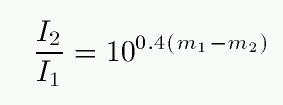Exercises:
1. One of the stars in the handle of the Big Dipper is really a pair of stars, Alcor and Mizar, just far enough apart for people with good eyes to distinguish. Given magnitudes of 4.00 for Alcor and 2.06 for Mizar, how many times brighter is Mizar than Alcor?

2. What is the ratio of intensities for a pair of stars 5 magnitudes apart? 10 magnitudes? 15 magnitudes? 20 magnitudes? Is there a simple rule for calculating these intensity ratios quickly?

3. The average diameter of the dark-adapted pupil in a human eye is about 6 millimeters; the average person can see a star of magnitude 6 on a clear, dark night. If the same person were to look through typical 7x35 binoculars, how faint a star might he be able to detect?

#### A very handy coincidence

Despite their arcane definition, magnitudes can occasionally be very quick and handy to use in practice. For example, one often looks for small changes in the brightness of a star or asteroid. A common way to describe small changes is in terms of percentages: "alpha Orionis faded by 3 percent over the past week." It turns out that there is a simple relationship between small percentage changes in intensity and the corresponding changes in magnitude:

```    if
a star changes its intensity by   N     percent,

then
its magnitude changes by about  0.01*N  mag.
```

For example, if alpha Orionis fades by 3 percent, then its magnitude increases by about 0.03 mag.

This rule is accurate to about ten percent -- the real change corresponding to fading by 3 percent is about 0.033 mag, not 0.030 mag. But under most circumstances, it's good enough. It works best for very small changes; applying it to changes greater than 15 or 20 percent yields results which are increasingly incorrect.

Exercise:
1. Using the definition of magnitudes given above, derive this relationship. Hint: look up the series expansion for e-to-the-x, when x is much less than 1.

#### The painful side of magnitudes: adding and subtracting

There are drawbacks to the magnitude system. One of the big ones is the work one must do when trying to figure out the result of adding or subtracting two stellar sources, rather than multiplying or dividing them. Suppose there are two stars, A and B, with magnitudes m(A) and m(B), which appear so close together that their light blends into a single source. What is the magnitude of the resulting blend?

```      m(A + B)  =?    m(A) + m(B)               NO!
```

The proper way to do this calculation is to convert the magnitudes back into intensities, add together the intensities, and then convert back into magnitudes. There's no way around it.

Exercise:
1. My eyesight is so poor that I can't distinguish Alcor from Mizar without my eyeglasses. Given magnitudes of 4.00 for Alcor and 2.06 for Mizar, what is the magnitude of the single blurry object I see?

#### Apparent and absolute magnitudes

When we look up into the sky at night, we see some stars which appear very bright, and others which are so faint that we can barely detect them.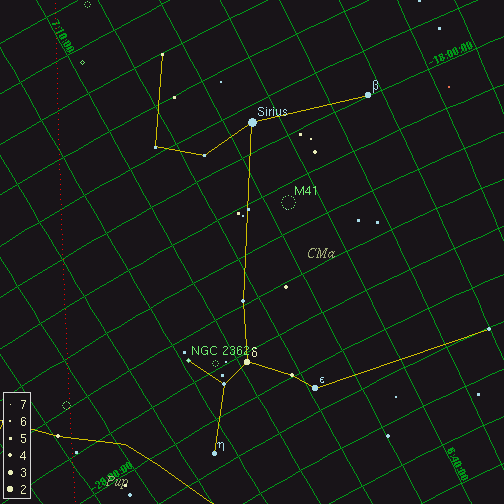The star Sirius, for example, has a magnitude of about -1.5; just a few degrees away, the star iota Canis Majoris shines feebly at magnitude 4.4.

```
Q:  How many times brighter does Sirius appear?
```
Does this mean that Sirius is a much more powerful star, one which emits hundreds of times as much energy as iota CMa?

No!

The reason, of course, is that two factors determine the apparent brightness of a star in our sky.

• The amount of light it emits
• Its distance from the Earth

In this particular instance, the apparent magnitude of these two stars, based on their apparent brightness is quite misleading. It turns out that Sirius has a parallax of 0.379 arcsec, whereas iota CMa has a parallax of only 0.001 arcsec.

```
Q:  How much farther away is iota CMa?
(yes, this is very uncertain)

Q:  If you include the distances of the
two stars, which one must be more luminous?

```

Since we often want to compare the intrinsic properties of stars, we'd like to have some measure of brightness which correlates directly with luminosity; a type of magnitude which does not depend on distance. The absolute magnitude of a star is defined as the apparent magnitude it would have, if it were moved to a distance of 10 parsecs from the Sun.

```
Q:  What is the absolute magnitude of Sirius?
What is the absolute magnitude of iota CMa?
```

The ordinary convention is to write apparent magnitudes with a lower-case letter m, and absolute magnitudes with an upper-case M. One can derive a formula which connects the apparent and absolute magnitudes of a star, using the inverse square law.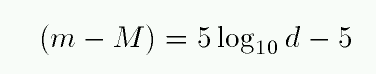```
Q:  How sensitive is the absolute magnitude to
an error in its distance?  For example,
a star with a 5 percent error in its distance
has what percentage error in its absolute
magnitude?

E.g.        m  = 6.0
true   d  = 100 pc
meas   d' = 105 pc

```

#### The distance modulus

The difference between the apparent and absolute magnitude of a star, (m - M), is called its distance modulus. As the equation above shows, it is a simple function of the distance to the star. In practice, astronomers sometimes prefer to specify the distance to a star by its distance modulus, rather than by the distance itself. Look at the abstract to this paper on the distance to stars in the Large Magellanic Cloud: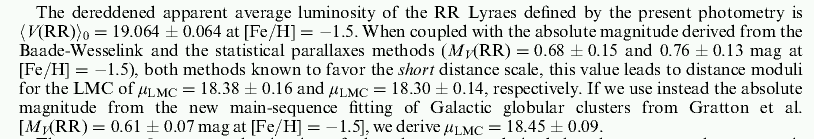Why use distance modulus instead of distance? I can think of two reasons, though they really boil down to the same thing.

1. Often, one determines the distance to a distant object by assuming that it is identical to some nearby object whose distance is known, and comparing the apparent brightness of the two objects. In this case, the quantities actually observed are the two apparent magnitudes; so using distance modulus is natural.

2. When planning an observing run, astronomers need to figure out how long their exposures should be. This depends on the apparent magnitude of their targets. If (as is often the case) an astronomer has a good idea of the intrinsic properties of target stars -- their absolute magnitudes -- he can most quickly and easily calculate their apparent magnitudes if he knows the distance modulus.

The basic idea is that, from a practical, observational point of view, it is often more useful to have a catalog of distance modulus values instead of real distances.

A second advantage appears when astronomers use distance modulus as a relative measure between two objects. For example, the Large Magellanic Cloud (LMC), the nearest galaxy to our own Milky Way, is often used as a stepping-stone to other, more distant galaxies. Our current estimate of the distance to the LMC is about 50 kpc. Suppose that you measure the distances to the LMC and several other galaxies by observing a particular sort of star in each galaxy. You might find

```            mag of star   distance mod       distance
galaxy        m        relative to LMC       (kpc)
------------------------------------------------------
LMC         16.5           --                50
M31         24.3           7.8             1800
M81         26.8          10.3             5740
------------------------------------------------------
```

Ten years from now, astronomers discover a systematic error in measurements to the LMC; instead of being 50 kpc away from us, it actually turns out to be 60 kpc away.

• if you published your results for M31 and M81 using the absolute distances for each one, in kpc, then your work immediately becomes invalid. In order to use your results, astronomers will have to re-calculate all your values using the new distance to the LMC
• but if you published your results in distance modulus, relative to the LMC, your results are still as correct as ever. Astronomers will still know exactly how much fainter a star in M31 will appear, relative to an identical star in the LMCCopyright © Michael Richmond. This work is licensed under a Creative Commons License.